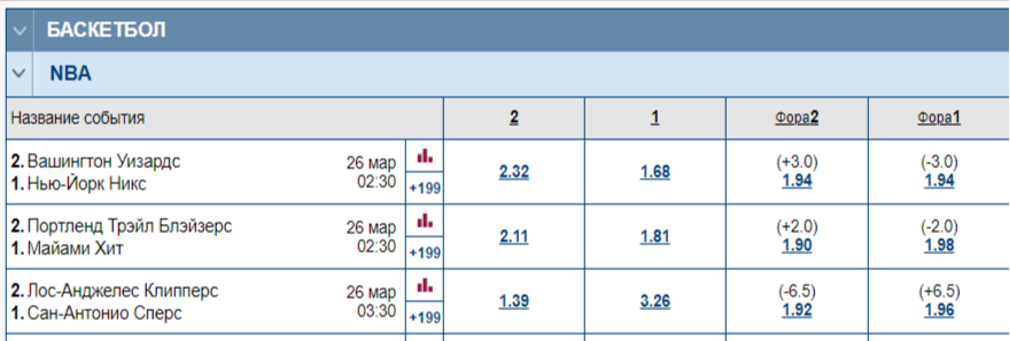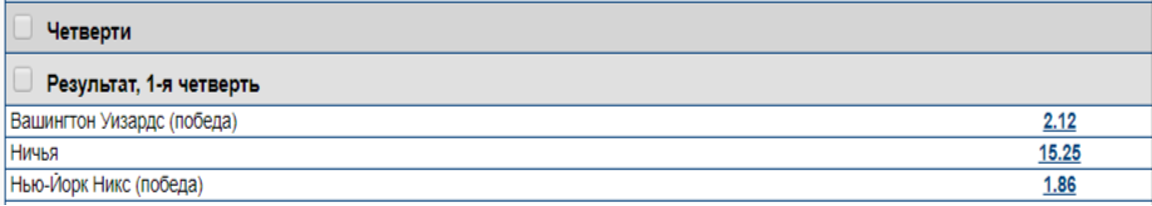What do the coefficients in the sports betting

30.05.2020

A newbie who visits a bookmaker's website for the first time often does not understand these strange numbers next to each outcome. These are the odds, by them you can understand a lot about the match, the outcomes, and the bookmaker itself.

Types of odds in bookmakers

The format of the odds differs depending on the geography. In the US, bookmakers use American odds, in Asian countries, their format, and in the UK they generally use old-fashioned fractions. In Europe and the CIS countries, the decimal format of odds is the most common.Numbers 2.32, 1.68, 1.94 and so on - this is the outcome rate

What information do the coefficients contain?

According to European odds, four main parameters can be understood:

1. Amount of payout for a bet on this odds;
2. Net winnings (profit) on a bet;
3. An approximate estimate of the probability of an outcome by the bookmaker;
4. Bookmaker's margin.

Payout amount

The calculation is simple. You need to multiply the offered odds by the amount of your bet, as a result, you get the amount of payment for the bet. For example, our rate is 3,500 rubles on the victory of “Washington” at a coefficient of 2.32. Let's translate it into rubles:

3,500 x 2.32 = 8,120 rubles will be paid by the bookmaker from the bet

If the bet itself (3,500) is deducted from the payment amount, then the net winnings (profit) for this bet is obtained, in our case, +4,620 rubles from the bet.

Approximate probabilities

The bookmaker does not take odds out of thin air, they reflect the approximate probability of a given outcome. If we take the conditional match between “Real” and “Torpedo”, the victory of the former will be assessed by a very small coefficient. And so always, the higher the probability of the outcome, the lower the odds on it.

Accordingly, we can conclude that the bookmakers believe that the Knicks' victory in the match with Washington is more likely, because the odds for them below. Moreover, we can calculate the odds based on the odds.

To do this, you need to divide 100% by the outcome coefficient

100 / 1.68 = 59.52% - the bookmaker estimated the odds of the bet on “winning 1”

100 / 2.32 = 43.1% - the bookmaker estimated the odds of the bet on “winning 2”

You ask: "How is it, 59.52% + 43.1% = 102.62%, how can there be more than 100% probability?" It is for this reason that the assessment of the probability of outcomes is approximate, because the coefficient also includes the bookmaker's commission for the provision of services.

Bookmaker margin

This is what all the bookmakers in the world profit from. The higher it is, the lower the coefficients in this bookmaker. In an ideal world, the odds for equiprobable outcomes would be 2.00. But in reality, this almost never happens. Some bookmakers give 1.90 in both directions, others even less.

How do I calculate the margin?

It is easy to find a convenient bookmaker's margin calculator in the public domain, but you can also calculate it manually using a simple calculator:

Divide 100% by the odds of each outcome, add the results and subtract one hundred from them

How it looks in our example, where there are only two possible outcomes:

(100 / 1,68 + 100 / 2,32) - 100 = 2,62%

This is the margin of the Marathon bookmaker on the outcome of this match. It can be very different depending on the office, the type of sport, the scale of the competition, the time remaining until the game. And even within the framework of one match it can fluctuate several times.

Let's calculate the margin on the outcomes in the first quarter, taking into account the draw:(100 / 2,12 + 100 / 15,25 + 100 / 1,86) - 100 = 7,49%

Here the commission is already much higher, but this is the same match and the same bookmaker. Obviously, when betting on an additional list, the ROI (an indicator of the efficiency with which a player makes bets) will suffer greatly.

What else you need to know about margin?

1. Firstly, the lower the average commission in the bookmaker, the more profitable it is to make bets with them in the future. After all, in fact, these are real rubles, by which each of our winnings decreases. Bookmakers offer a margin of 0.5% to 15%, and with 10,000 rubles of a win it is much more profitable to pay 50 rubles than 1,500.
2. Secondly, it is more profitable to bet on a match based on the main outcomes, and not on an additional list where the coefficients are consistently worse. In live, the commission is almost always higher than it was before the game. At major tournaments, it is lower than at minor ones. The markets you choose to bet have a very strong impact on distance ROI.

***

To summarize: the best odds are provided by the big bookmakers on the most popular sports and the biggest tournaments. Better to bet on the main line, which is offered for the match (wins, handicaps, totals) literally a few hours before the event - at this time you can place bets with almost zero margin.

***

Conclusion

Just a couple of numbers can give us so much useful information. Having made several calculations, we can understand not only what kind of payoff we will get by placing bets on this coefficient, but also the net winnings from the bet.

The probabilities of the selected outcomes, what are our chances of winning, and even how much the selected bookmaker is remotely beneficial. Love sports, learn how to place your bets correctly and calculate every number - even hundredths at a distance are of great importance for a bettor's ROI.